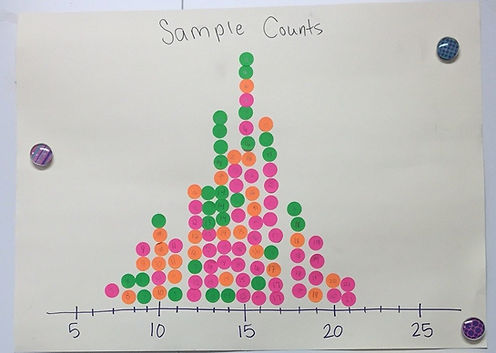## Chapter 6 - Day 4 - Lesson 6.3

##### Learning Targets
• Calculate the mean and the standard deviation of the sampling distribution of a sample count and interpret the standard deviation.

• Determine if the sampling distribution of a sample count is approximately normal.

• If appropriate, use the normal approximation to the binomial distribution to calculate probabilities involving a sample count.

##### Activity: Is this a fair coin?

Lesson 6.3 introduces the idea of a sample count.  You’ll need coins, a poster, stickers and table A for this activity.  We begin with a quick review of binomial random variables which were covered in lesson 5.4.  It’s so beneficial to students to connect prior learning with new learning.  We do this not only for review but also to make students feel confident, like what they are about to learn won’t be that hard.

Students should flip their coin 30 times and keep track of the number of heads.  When they’re done, they’ll write the number they got on a sticker and add it to the dotplot on the board.  They’ll do this 4 times.After students collect their data and add their stickers to the poster, revisit question #4 and have a discussion about what the sampling distribution would look like if we did this many, many times.  Students should reason that it will begin to look more and more normal.  But how do we check if a sampling distribution of sample counts will be approximately normal?  Explain Large Counts to students and add it to the margins of the activity.

We made formula review sheet for this chapter to help students keep the different formulas for sample counts, proportions or means.  We will fill it out each day.

##### Teaching Tip:

If you teach AP Stats, you’ll notice that this lesson is a nice stepping stone for students to get to sample proportions.  This lesson really ties first semester to second semester really nicely.  We’re able to connect with the binomial mean and standard deviation formulas, z-scores, normal curve calculations and the idea of convincing evidence.